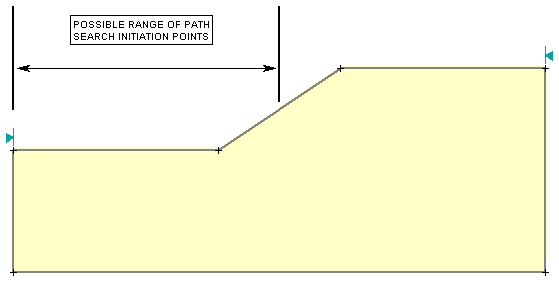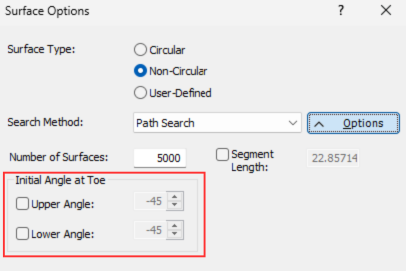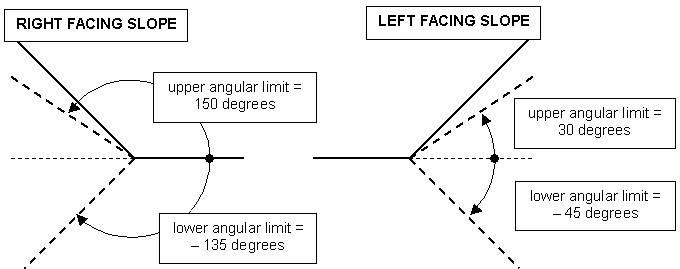Join one of our upcoming courses taking place around the world! Explore now

# Path Search

A Path Search is one of the Search Methods which can be used in Slide2 for locating the Global Minimum safety factor for NON-CIRCULAR slip surfaces. In order to perform a Path Search, the Surface Options dialog must be configured as follows:

• Surface Type = Non-Circular
• Search Method = Path Search

## How Does the Path Search Work?

The Path Search in Slide2 generates random NON-CIRCULAR (piece-wise linear) slip surfaces as follows:

1. For each slip surface, a starting point is randomly generated on the slope surface. The allowable range for the starting point, is defined by the Slope Limits, as follows:
• If a single set of Slope Limits is defined, then Slide2 will automatically divide the range in half, and use the range closest to the toe of the slope, to generate the slip surface starting points.
• If a double set of Slope Limits is defined, then Slide2 will use the range closest to the toe of the slope to generate the slip surface starting points.
A double set of Slope Limits is recommended for use with the Path Search in Slide2, as it allows you to more accurately define the initiation range for slip surfaces. See the Define Limits topic for information about how to customize the Slope Limits in Slide2.

These two cases are illustrated below, for a Right to Left Failure Direction.

Possible range of Path Search Initiation points, for SINGLE set of Slope Limits.Possible range of Path Search Initiation points, for DOUBLE set of Slope Limits.1. Once a slip surface initiation point has been generated, Slide2 then generates the FIRST segment of the non-circular slip surface, according to the Initial Angle at Toe and the Segment Length specified in the Surface Options dialog for the Path Search.
2. See below for details about the Path Search Options.
3. The remainder of the slip surface is then generated according to the Segment Length, and the Path Search algorithm.
4. The endpoint of each slip surface must intersect the slope surface within the Slope Limits. The Slope Limits DO NOT influence the location of the endpoint, but are used as a filter, to ensure that the endpoint is within the Slope Limits. If the endpoint is NOT within the Slope Limits, the surface is discarded.
5. Slide2 will repeat steps 1 to 4, until the number of valid slip surfaces generated is equal to the Number of Surfaces specified in the Path Search Options.
• the Path Search algorithm used in Slide2 is modeled after the "Irregular Surface Search" used in the slope stability program XSTABL. A few modifications have been made, but the basic methodology is the same.
• Unlike the Grid Search (for Circular Surfaces) or the Block Search (Non-Circular Surfaces), the Path Search DOES NOT REQUIRE ANY SEARCH OBJECTS TO BE DEFINED. The Path Search is entirely defined by the Path Search Options specified in the Surface Options dialog, and the current Slope Limits.

## Path Search Options

The following Path Search Options are defined in the Surface Options dialog.

## Number of Surfaces

This is the total number of valid surfaces generated by the Path Search.

Invalid surfaces generated by the Path Search are discarded, and are NOT included in this number. Invalid surfaces may occur if the slip surface endpoint does not intersect the slope within the Slope Limits, as described above, or other factors may be involved (e.g. incorrect Initial Angle at Toe).

## Initial Angle at Toe

The Initial Angle at Toe is the orientation of the FIRST line segment of a slip surface generated by the Path Search.

Slide2 ALWAYS generates slip surfaces for the Path Search, starting at the toe of the slope, and progressing towards the crest, regardless of the Failure Direction (Right to Left or Left to Right).By default, Slide2 randomly generates the Initial Angle at Toe, for each slip surface, within the following angular limits:

Upper Angle – the default upper angular limit depends on the angle of the slope segment at the initiation point of the slip surface, and is equal to ( b – 5 ) degrees, where b is the angle of the slope segment.

Lower Angle – the default lower angular limit is 45 degrees below the horizontal.

Upper and lower angular limits for first segment of slip surfaceUser defined angular limits can be specified by selecting the Upper Angle or Lower Angle checkboxes, and entering the desired angular limits.

The angle measurement convention is the usual convention used in Slide2, where a positive angle is measured in degrees COUNTER-CLOCKWISE from the positive horizontal axis.
Because of the angular measurement convention, the values of user defined angular limits will depend on the Failure Direction. For example: an Upper Angular Limit of 30 degrees for a Right to Left Failure Direction, is equivalent to an Upper Angular Limit of 150 degrees for a Left to Right Failure Direction. This is illustrated in the figure below.

Example of equivalent angular limits for left and right facing slopes.## Segment Length

This is the length used by Slide2 for each segment of a non-circular surface generated by a Path Search. NOTE:

• Slide2 automatically calculates a suitable default Segment Length, based on the extents of the model boundaries. It is NOT necessary for the user to specify a Segment Length, unless they wish to use a specific number.
• A user-defined Segment Length can be specified, by selecting the Segment Length checkbox and entering a Segment Length in the edit box.
• If the Segment Length is too small, invalid surfaces may result, if the surface becomes "too irregular" (i.e. kinematically inadmissible), or if the total number of segments generated for a surface exceeds the Number of Slices entered in the Project Settings dialog.
• A recommended Segment Length which should generate valid slip surfaces in the majority of cases is approximately 0.3H, where H is the maximum height of the slope.

## Convex Surfaces Only

See the Block Search topic for a description of the Convex Surfaces Only option, as the effect on both the Block Search and the Path Search is the same.

## Optimize Surfaces

A supplementary optimization can be applied to slip surfaces, after the initial analysis, in order to locate slip surfaces with an even lower factor of safety. See the Optimize Surfaces topic for details.

Surface Filter

See the Surface Options topic for details about the Minimum Elevation, Minimum Depth, Minimum Area, Minimum Weight slip surface filter options.

Random Number Generation

The generation of slip surfaces by the Path Search is based on the generation of random numbers. For the Path Search, random numbers are used to generate:

• the slip surface initiation points
• the angle of the first slip surface segment
• the angle of all subsequent slip surface segments

The method of generating random numbers is determined by the Random Numbers option in the Project Settings dialog. There are two methods available, Random or Pseudo-Random. Pseudo Random generation uses a constant seed value to generate random numbers. For a given model, this will generate exactly the same slip surfaces each time the analysis is run, and therefore the results will be reproducible. Random generation uses a variable seed value. This will generate DIFFERENT surfaces each time the analysis is run, and therefore results will be different each time Compute is selected. For details about the Random and Pseudo Random options, see the Random Numbers topic.